## The CDF Canada group is involved in the following research projects:

### Top Quark Physics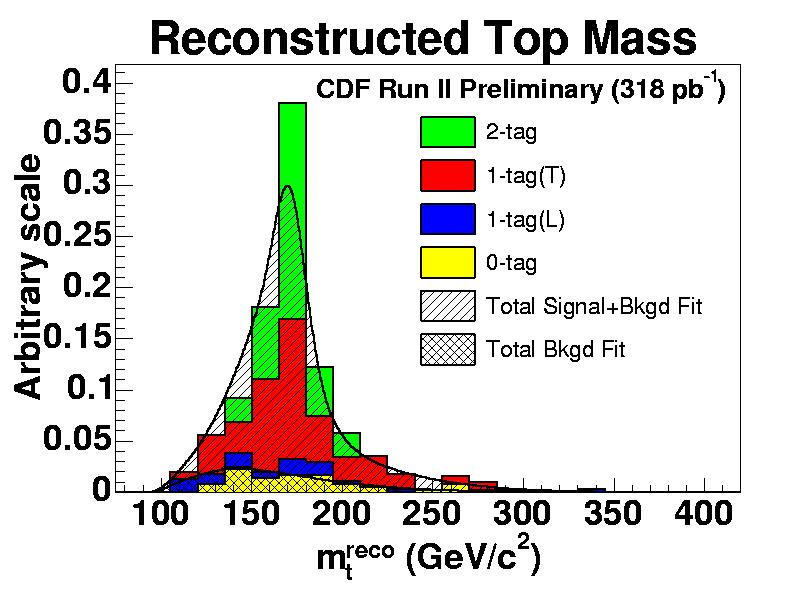#### Top Quark Mass with In Situ Determination of the Jet Energy Scale

The top quark mass is a fundamental parameter of the Standard Model, and it, along with the W boson mass, constrains the Higgs boson mass. The mass is measured by selecting ttbar events where one W boson decay leptonically and the other hadronically. The observed invariant mass of the hadronic W boson decay is used to reduce the largest systematic uncertainty arising from the jet energy scale. The top quark mass and hadronic W boson mass distributions reconstructed in data are compared to Monte Carlo expectations to determine simultaneously the top quark mass and the jet energy scale. We measure M_top = 173.5 +3.7/-3.6 (stat.+JES) +/- 1.3 (syst.) GeV/c^2, where the first uncertainty includes both the statistical and the jet energy scale (JES) uncertainty. This constitutes the best measurement of the top quark mass to date.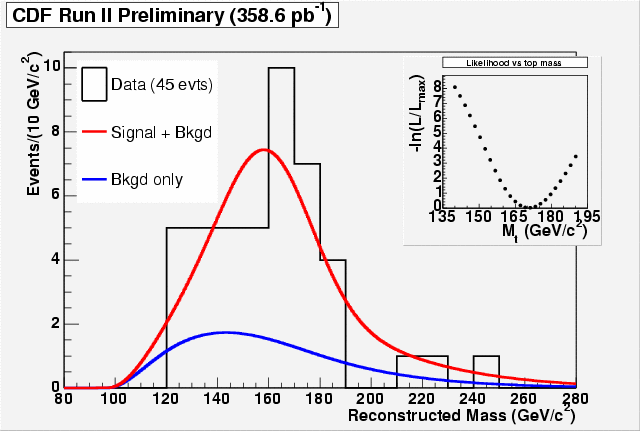#### Top Quark Mass Measurements in the Dilepton Channel using the Neutrino Weighting Algorithm

The measurement of the top quark mass (with the measurement of the W boson mass) constrains the prediction of the Higgs boson mass. If the Higgs boson is discovered, this will allow a self-consistency test of the Standard Model. To measure of the Top Quark Mass we select top candidates that are consistent with two W bosons decaying to a charged lepton and a neutrino. Only one of the two charged leptons is required to be identified as an electron or a muon candidate, while the other is simply a well measured track. We use an algorithm that weighs each possibility of neutrino direction to reconstruct a top quark mass in each event. We compare the resulting distribution to Monte Carlo templates to obtain the top quark mass.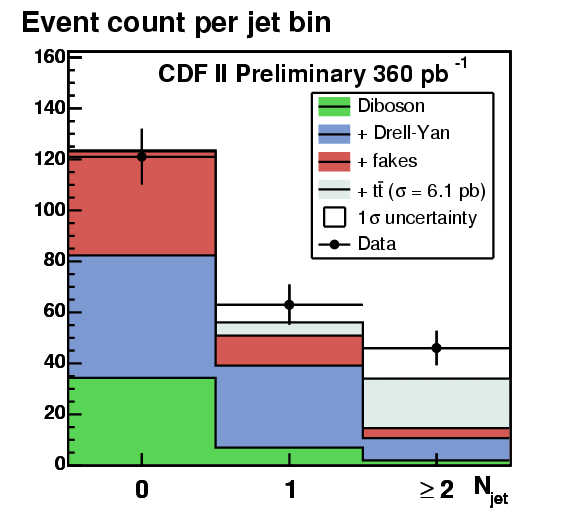#### Top Quark Pair Production Cross Section using Lepton + Isolated Track Selection

Top decays via the dilepton channel have fewer background counts compared to other channels. But this channel suffers from a low branching ratio, which limits its statistical power. The lepton + isolated track selection increases acceptance to single prong hadronic tau decays, and leptons in uninsturmented regions of the detector by requiring a well measured and energetically isolated track and a traditionally identified lepton instead of two leptons.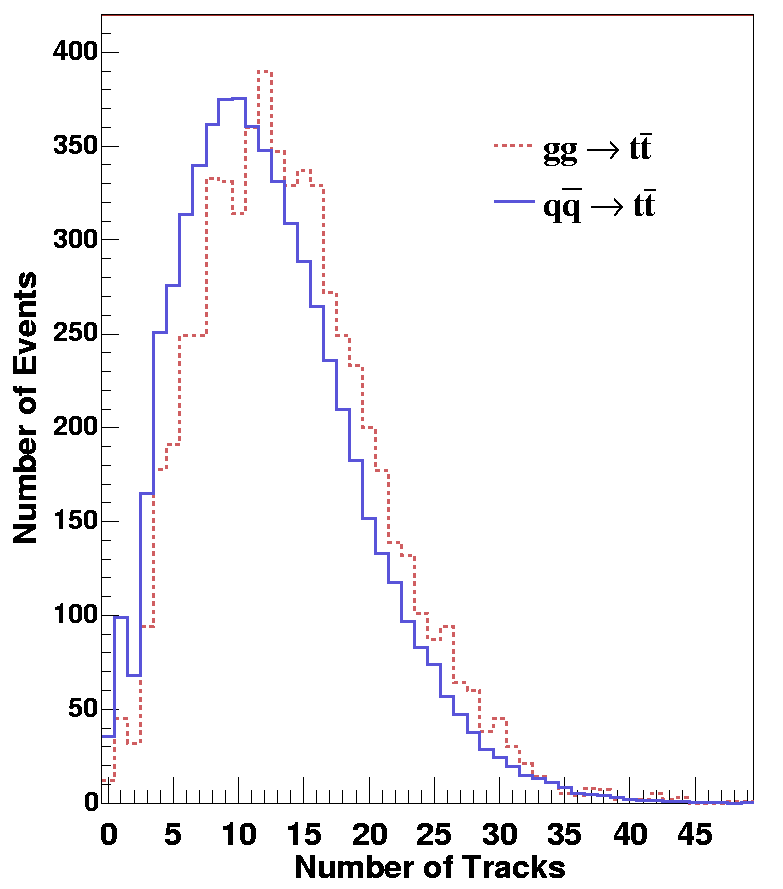#### Study of Top Quark Pair Production Mechanisms

Top quark is the heaviest elementary particle observed and is perceived as an important probe to understand the origin of the mass. Since its discovery in 1995, there have been many studies to measure its mass, its production rate and its other properties. According to the Standard Model, top quarks are dominantly produced in pairs. The rate for the top quark pair (ttbar) production have been measured, but the rate for ttbar production has not been broken up to the rate for different mechanisms. Our analysis is the first measurement of the contribution of gluon-gluon fusion (gg) to produce top quark pairs over the contributions through quark-antiquark annihilation (qqbar). In order to make this measurement, we must distinguish between the two different processes for ttbar production. We take advantage of the fact that it is more likely for gluons to radiate a gluon than it is for quarks to do so. Therefore, we expect to see more particles in gg events than in qqbar events. We use the distribution of low energy charged particles to find the ratio of ttbar production through gg over ttbar production through qqbar.

### Electroweak Physics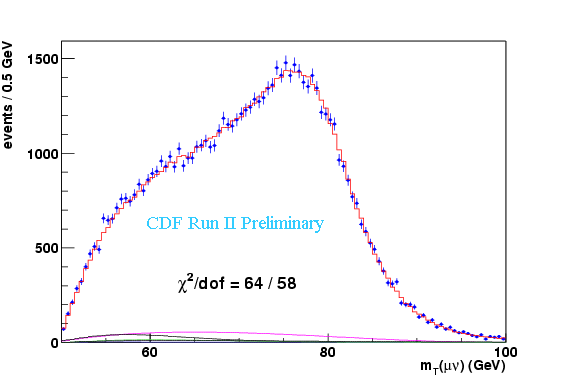#### W Boson Mass

The W boson mass is a fundamental parameter of the Standard Model. Together with the top quark mass, the W boson mass constrains the Higgs boson mass, which has not yet been observed experimentally. We measure the W boson mass in the two clean leptonic decay channels W->mu nu and W->e nu. The signature is a high energy lepton with large missing transverse energy originating from the neutrino, which does not interact with the detector. The momentum balance in the direction of the beam is unconstrained and as a result, we study the W events in the plane transverse to the beam. A typically used quantity is the transverse mass, mT, which is similar to the invariant mass, just in the two transverse dimensions. There are two important aspects to a precise W boson mass measurement. Firstly, calibrating the detector to the highest possible level and secondly a detailed simulation, including all relevant W production kinematics and detector effects.

### QCD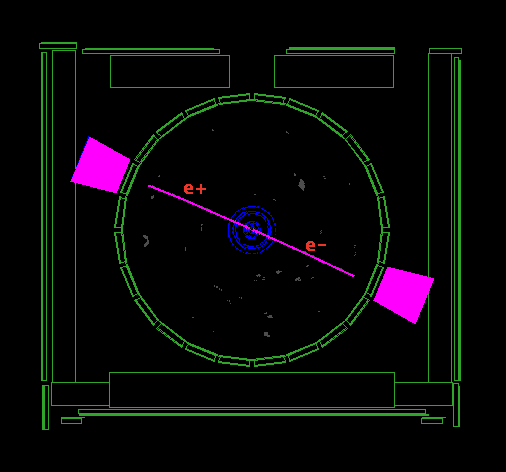#### Search for Exclusive Processes

There are Standard Model processes in which hadrons do not dissociate in the interaction. Without hadron dissociation there is no underlying event in the interaction - only a very clean, exclusive, central system is observerd in the detectror. We are searching for two exclusive processes; double pomeron exchange to two photons, and photo-production of electron pairs. Theoretically, the pomeron process can produce a Higgs Boson. If suitable detectors are installed at the LHC, the outgoing protons can be used like a spectrometer to measure the Higgs mass. Because the photo-production process is governed by QED its cross section can be accurately calculated, meaning that it could be a useful tool to measure the luminosity at the LHC. Before either of these processes can be used at the LHC, they must be understood here at the Tevatron - the goal of this research is to observe and understand these unique events at CDF.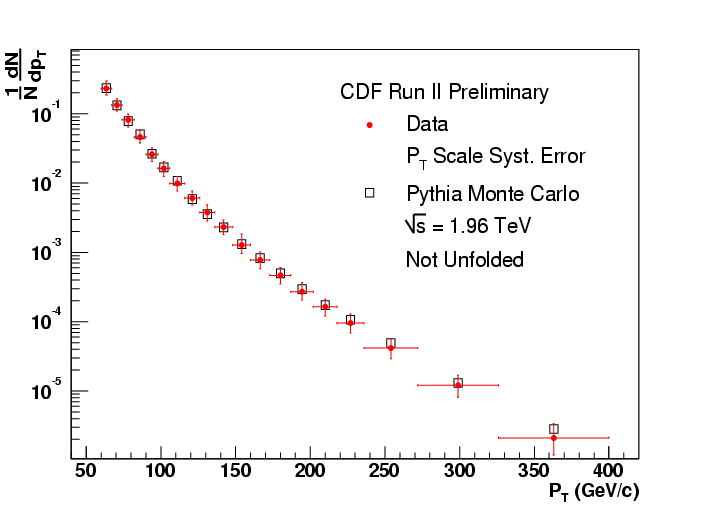#### Measurement of the Inclusive b-Jet Cross Section

The notion and discovery of "quarks" has revolutionized humankind's understanding of physics at the most fundamental level. The "strong" force that binds quarks together is described by the theory of Quantum ChromoDynamics (QCD). In this analysis we test QCD by measuring the production of bottom (b) quarks in highly energetic jets resulting from proton-antiproton collisions at the Fermilab Tevatron, using the CDF-II detector. The term "jet" refers to a spray of particles traveling in roughly the same direction, all originating from a high-energy parent particle. To detect b-quark jets we rely on the excellent particle tracking resolution of CDF-II and an advanced "Jet Probability" analysis algorithm to determine if the collection of particle tracks within a jet are consistent with the decay of a b-quark. We compare our measurement to the predictions of QCD theory.

### Exotic Physics#### Seach for Supersymmetry in Missing Transverse Energy and Multi-jet Events

Supersymmetry (SUSY) is one of the most popular candidates for physics beyond the Standard Model. In supersymmetric theories, each Standard Model boson has a fermionic partner, and vice versa. The gluon's partner is called a "gluino", and the partners of the quarks are called "squarks". In one range of SUSY theories, where the quantity R-parity is conserved, pair-produced squarks and gluinos would decay, producing energetic hadronic jets and weakly interacting stable "neutralinos". These neutralinos would escape detection by the CDF calorimeter, thus producing missing transverse energy (MET). In this search, a signal region with high MET and three or more energetic jets is defined. The signal region is treated as a "blind box", and will not be examined until the backgrounds to the signal are fully understood in nearby control regions and the cuts on the data are optimized. As CDF has collected more than four times the data so far in its second run as it did in its first run, when a similar search was performed, analysis of this data should extend existing limits on the squark and gluino masses, and thus on the fundamental SUSY parameters.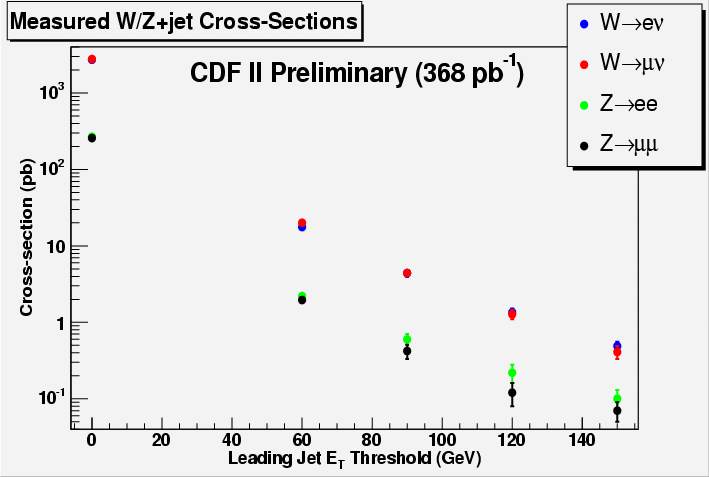#### Search for Extra Dimensions in Jets + Missing Energy in Run II

In order to make sense of many fundamental aspects of the Universe such as the quantum description of gravity, the origin of the mass of particles or the the possible compositness of leptons and quarks, the so far successful Standard Model (SM) of particle physics needs to be extended. The observation of an excess over the SM expectation in events with a mono-jet plus large missing transverse energy topology would provide a clear signal of possible new physics. In order to find such an excess, we first need to estimate the number of Z(->nunu)+1-jet events that will be contained in our data sample. This is deduced from the number of Z->ee, Z->mumu, W->enu and W->munu plus 1-jet events that we count. We expect (including some less important background events) a total of 265+/-30 SM events in our data sample and we observe 263. Thus no excess has yet been found. From this we set a limit on the possibility of observing a quantum of gravity (graviton) by setting a lower limit on the Planck scale of a D-dimensional world (D in [6,10]).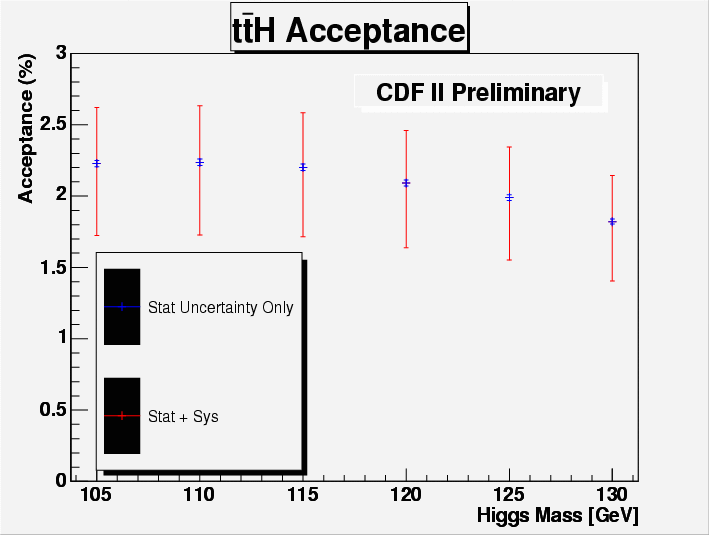#### Search for Higgs Boson in ttH Events

Though predicted by the Standard Model as the key to electroweak symmetry breaking, the Higgs boson has yet to be observed. The interaction strengths of the Higgs boson with other particles is proportional to their mass. Thus the channel ttH provides an interesting search channel for the Higgs boson and additionally allows us to understand the mechanism that gives mass to particles. Sensitivity to ttH requires lepton identification and efficient identification of jets originating from b-quarks.